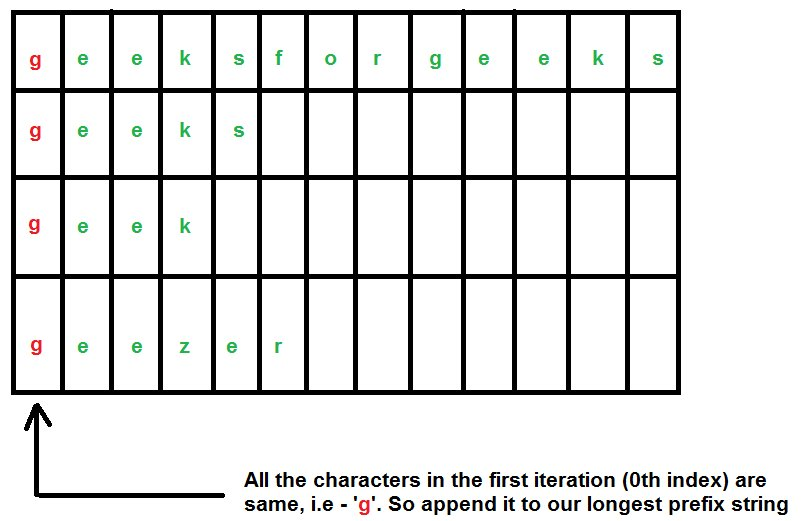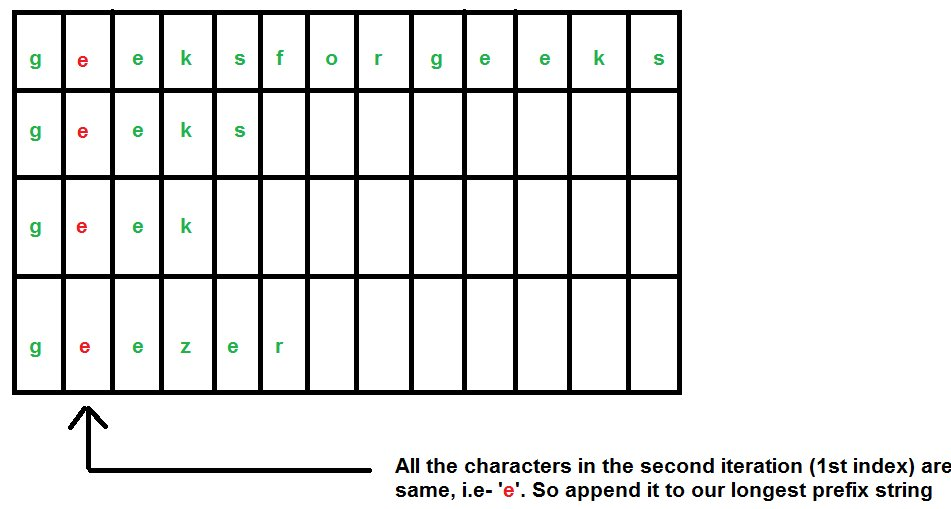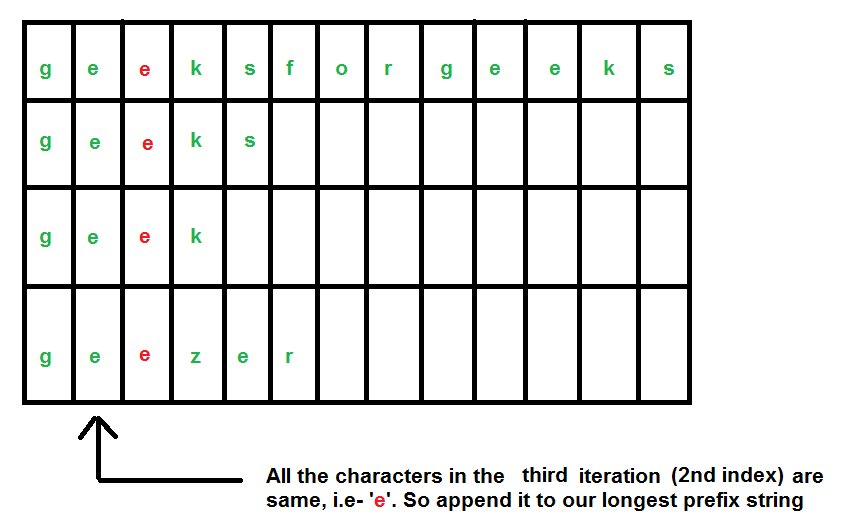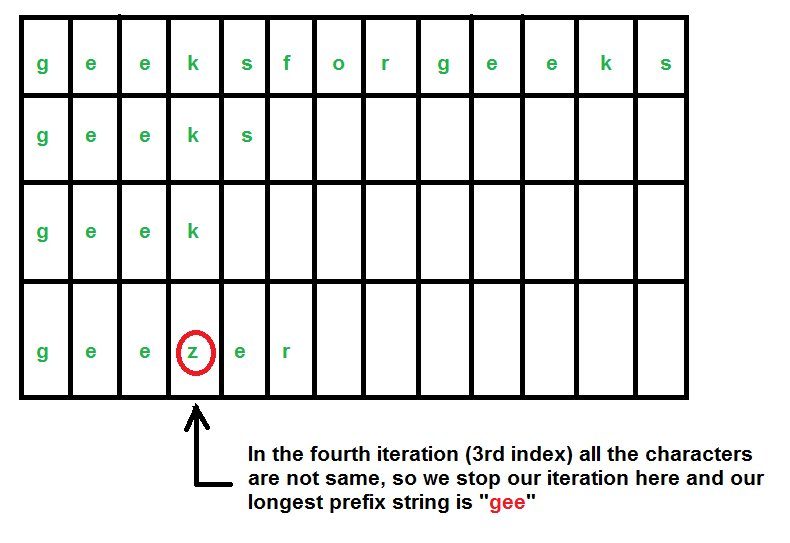# Longest Common Prefix using Character by Character Matching

• Difficulty Level : Easy
• Last Updated : 15 Jun, 2022

Given a set of strings, find the longest common prefix.

```Input  : {“geeksforgeeks”, “geeks”, “geek”, “geezer”}
Output : "gee"

Input  : {"apple", "ape", "april"}
Output : "ap"```

We have discussed word by word matching algorithm in previous post.
In this algorithm, instead of going through the strings one by one, we will go through the characters one by one.
We consider our strings as – “geeksforgeeks”, “geeks”, “geek”, “geezer”.Below is the implementation of this approach.

## C++

 `//  A C++ Program to find the longest common prefix``#include``using` `namespace` `std;` `// A Function to find the string having the minimum``// length and returns that length``int` `findMinLength(string arr[], ``int` `n)``{``    ``int` `min = arr.length();` `    ``for` `(``int` `i=1; i

## Java

 `// A Java Program to find the longest common prefix``class` `GFG``{` `    ``// A Function to find the string having the minimum``    ``// length and returns that length``    ``static` `int` `findMinLength(String arr[], ``int` `n)``    ``{``        ``int` `min = arr[``0``].length();` `        ``for` `(``int` `i = ``1``; i < n; i++)``        ``{``            ``if` `(arr[i].length() < min)``            ``{``                ``min = arr[i].length();``            ``}``        ``}` `        ``return` `(min);``    ``}` `    ``// A Function that returns the longest common prefix``    ``// from the array of strings``    ``static` `String commonPrefix(String arr[], ``int` `n)``    ``{``        ``int` `minlen = findMinLength(arr, n);` `        ``String result = ``""``; ``// Our resultant string``        ``char` `current; ``// The current character` `        ``for` `(``int` `i = ``0``; i < minlen; i++)``        ``{``            ``// Current character (must be same``            ``// in all strings to be a part of``            ``// result)``            ``current = arr[``0``].charAt(i);` `            ``for` `(``int` `j = ``1``; j < n; j++)``            ``{``                ``if` `(arr[j].charAt(i) != current)``                ``{``                    ``return` `result;``                ``}``            ``}` `            ``// Append to result``            ``result += (current);``        ``}` `        ``return` `(result);``    ``}` `    ``// Driver program to test above function``    ``public` `static` `void` `main(String[] args)``    ``{``        ``String arr[] = {``"geeksforgeeks"``, ``"geeks"``,``            ``"geek"``, ``"geezer"``};``        ``int` `n = arr.length;` `        ``String ans = commonPrefix(arr, n);` `        ``if` `(ans.length() > ``0``) {``            ``System.out.println(``"The longest common prefix is "``                    ``+ ans);``        ``} ``else` `{``            ``System.out.println(``"There is no common prefix"``);``        ``}``    ``}``}` `// This code contributed by Rajput-Ji`

## Python 3

 `# Python 3 Program to find the longest common prefix`` ` `# A Function to find the string having the minimum``# length and returns that length``def` `findMinLength(arr, n):` `    ``min` `=` `len``(arr[``0``])`` ` `    ``for` `i ``in` `range``(``1``,n):``        ``if` `(``len``(arr[i])< ``min``):``            ``min` `=` `len``(arr[i])`` ` `    ``return``(``min``)`` ` `# A Function that returns the longest common prefix``# from the array of strings``def` `commonPrefix(arr, n):` `    ``minlen ``=` `findMinLength(arr, n)``    ``result ``=``""``    ``for` `i ``in` `range``(minlen):``    ` `        ``# Current character (must be same``        ``# in all strings to be a part of``        ``# result)``        ``current ``=` `arr[``0``][i]`` ` `        ``for` `j ``in` `range``(``1``,n):``            ``if` `(arr[j][i] !``=` `current):``                ``return` `result`` ` `        ``# Append to result``        ``result ``=` `result``+``current`` ` `    ``return` `(result)`` ` `# Driver program to test above function``if` `__name__ ``=``=` `"__main__"``:``    ` `    ``arr ``=` `[``"geeksforgeeks"``, ``"geeks"``,``                    ``"geek"``, ``"geezer"``]``    ``n ``=` `len``(arr)`` ` `    ``ans ``=` `commonPrefix (arr, n)`` ` `    ``if` `(``len``(ans)):``        ``print``(``"The longest common prefix is "``,ans)``    ``else``:``        ``print``(``"There is no common prefix"``)`

## C#

 `// A C# Program to find the longest common prefix``using` `System;``    ` `class` `GFG``{` `    ``// A Function to find the string having the minimum``    ``// length and returns that length``    ``static` `int` `findMinLength(String []arr, ``int` `n)``    ``{``        ``int` `min = arr.Length;` `        ``for` `(``int` `i = 1; i < n; i++)``        ``{``            ``if` `(arr[i].Length < min)``            ``{``                ``min = arr[i].Length;``            ``}``        ``}` `        ``return` `(min);``    ``}` `    ``// A Function that returns the longest common prefix``    ``// from the array of strings``    ``static` `String commonPrefix(String []arr, ``int` `n)``    ``{``        ``int` `minlen = findMinLength(arr, n);` `        ``String result = ``""``; ``// Our resultant string``        ``char` `current; ``// The current character` `        ``for` `(``int` `i = 0; i < minlen; i++)``        ``{``            ``// Current character (must be same``            ``// in all strings to be a part of``            ``// result)``            ``current = arr[i];` `            ``for` `(``int` `j = 1; j < n; j++)``            ``{``                ``if` `(arr[j][i] != current)``                ``{``                    ``return` `result;``                ``}``            ``}` `            ``// Append to result``            ``result += (current);``        ``}` `        ``return` `(result);``    ``}` `    ``// Driver code``    ``public` `static` `void` `Main(String[] args)``    ``{``        ``String []arr = {``"geeksforgeeks"``, ``"geeks"``,``            ``"geek"``, ``"geezer"``};``        ``int` `n = arr.Length;` `        ``String ans = commonPrefix(arr, n);` `        ``if` `(ans.Length > 0)``        ``{``            ``Console.WriteLine(``"The longest common prefix is "``                    ``+ ans);``        ``}``        ``else``        ``{``            ``Console.WriteLine(``"There is no common prefix"``);``        ``}``    ``}``}` `/* This code contributed by PrinciRaj1992 */`

## Javascript

 ``

Output

`The longest common prefix is gee`

Output :

`The longest common prefix is  gee`

How is this algorithm better than the “Word by Word Matching” algorithm ?-
In Set 1 we discussed about the “Word by Word Matching” Algorithm.
Suppose you have the input strings as- “geeksforgeeks”, “geeks”, “geek”, “geezer”, “x”.
Now there is no common prefix string of the above strings. By the “Word by Word Matching” algorithm discussed in Set 1, we come to the conclusion that there is no common prefix string by traversing all the strings. But if we use this algorithm, then in the first iteration itself we will come to know that there is no common prefix string, as we don’t go further to look for the second character of each strings.
This algorithm has a huge advantage when there are too many strings.
Time Complexity : Since we are iterating through all the characters of all the strings, so we can say that the time complexity is O(N M) where,

```N = Number of strings
M = Length of the largest string string ```

Auxiliary Space : To store the longest prefix string we are allocating space which is O(M).

Method 3(using some in-built C++ STL function):

In this approach first of all we will find the string with smallest length. Then we will search in all other strings if this smallest string is present in them as a prefix or not. If in all strings this small string is present is present then we will print this string else we will keep reducing the length of the smallest string by one until its length becomes zero.

Below is the implementation of the above Approach:

## C++

 `// C++ program to find the longest common``// prefix.``#include ``using` `namespace` `std;``// function to find the smallest string``// among all string``int` `shortest_string(string s[], ``int` `n)``{``    ``int` `minlength = INT_MAX, min_index;``    ``for` `(``int` `i = 0; i < n; i++) {``        ``if` `(s[i].length() < minlength) {``            ``minlength = s[i].length();``            ``min_index = i;``        ``}``    ``}``    ``return` `min_index;``}``// function to find longest common``// prefix among all strings.``string findprefix(string s[], ``int` `n)``{``    ``// index of the smallest string``    ``int` `shortest_string_index = shortest_string(s, n);` `    ``while` `(s[shortest_string_index].length() > 0) {` `        ``int` `count = 0;``        ``for` `(``int` `i = 0; i < n; i++) {``            ``// checking whether all strings have prefix``            ``// which is equal to smallest string``            ``if` `(s[i].find(s[shortest_string_index]) == 0) {``                ``count++;``            ``}``        ``}``        ``// checking that all the string's``        ``// prefix is equal to smallest string``        ``// or not.``        ``if` `(count == n) {``            ``cout << ``"longest common prefix is: "` `<< endl;``            ``return` `s[shortest_string_index];``            ``break``;``        ``}``        ``// deleting the last character``        ``// of the smallest string.``        ``s[shortest_string_index].pop_back();``    ``}` `    ``return` `"no common prefix among all strings"``;``}``// driver code``int` `main()``{``    ``string s[]``        ``= { ``"geeksforgeeks"``, ``"geeks"``, ``"geek"``, ``"geezer"` `};``    ``int` `n = ``sizeof``(s) / ``sizeof``(s);``    ``// function call``    ``cout << findprefix(s, n);``    ``return` `0;``}` `// this code is contributed by Machhaliya Mohammad.`

Output

```longest common prefix is:
gee```

Time complexity: O(n*m) where n is total number of strings and m is length of the smallest string among all strings.

Auxiliary space: O(1)

This article is contributed by Rachit Belwariar. If you like GeeksforGeeks and would like to contribute, you can also write an article and mail your article to review-team@geeksforgeeks.org. See your article appearing on the GeeksforGeeks main page and help other Geeks.